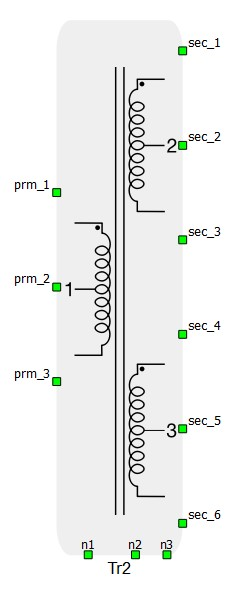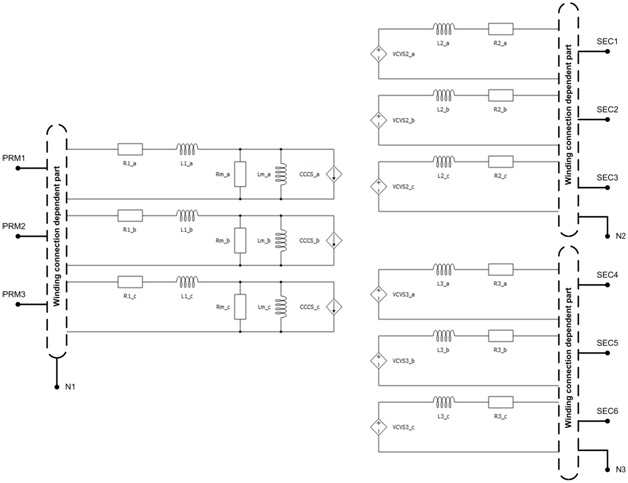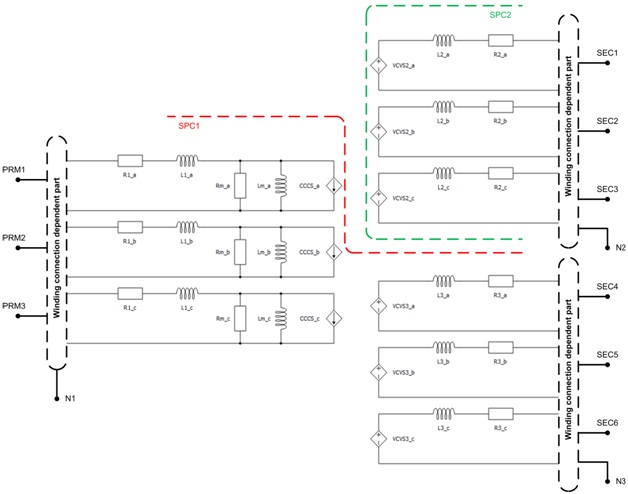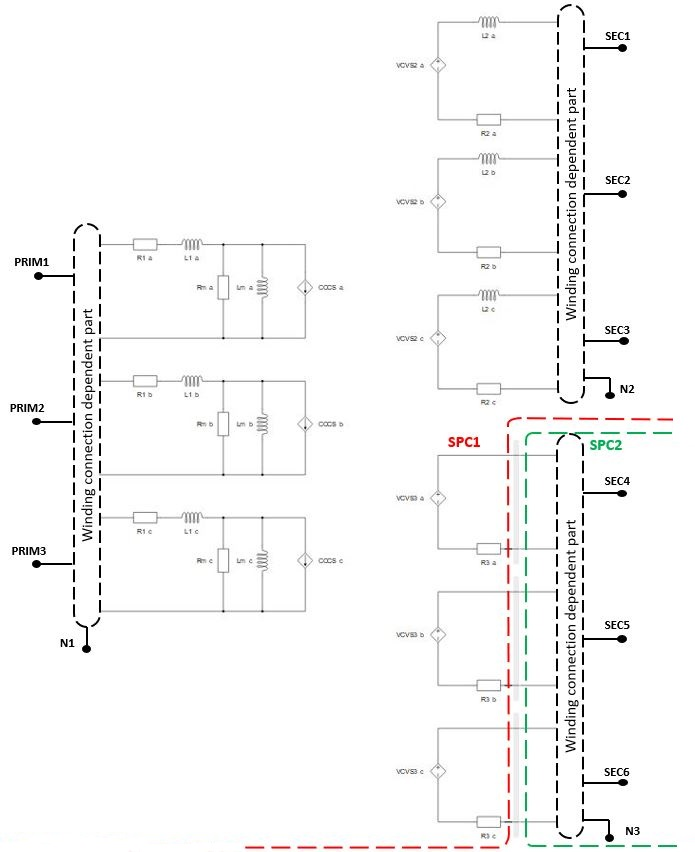# Three phase three winding transformer

This section describes three phase three winding transformer

Three-phase three winding transformer is modeled as three single-phase three winding transformers, meaning that only magnetic coupling between windings of the same phase are taken into account.

The magnetization inductance Lm can be linear or with saturation, it is modeled on the primary side of the transformer. Core losses are modeled as Rm resistance located on the primary side of the transformer. Also, it is possible to neglect Lm and Rm by selecting Lm/Rm neglected in the Core model property. For more informations, please refer to Core model

Schematic symbol and input parameters for three phase three winding transformer block are given in Table 1.

Table 1. Three phase three winding transformer component in HIL Toolbox
component component dialog window component parametersThree phase three winding transformer• Input parameters option (SI or p.u.)
• Nominal Power of the transformer in VA (Sn)
• Nominal frequency in Hz (fn)
• Nominal line to line voltage of winding x in volts RMS (Vx, x = 1, 2, 3)
• Resistance of winding x in ohms (Rx, x = 1, 2, 3)
• Leakage inductance of winding x in henries (Lx, x = 1, 2, 3)
• Core model - Linear, Non-Linear, Rm/Lm neglected
• Resistance representing core losses, in ohms (Rm)
• Magnetization inductance in henries (Lm)• Winding 1 connection (D or Y)
• Winding 2 connection (D or Y)
• Winding 3 connection (D or Y)
• clk_num_12 - determines the phase displacement between voltages of winding 1 and 2
• clk_num_13 - determines the phase displacement between voltages of winding 1 and 3• Embedded coupling 1-2 - inserts a coupling component (Ideal Transformer based or TLM) between winding 1 and 2.
• Embedded coupling 1-3 - inserts a coupling component (Ideal Transformer based or TLM) between winding 1 and 3.
• Type of coupling component - defines whether the inserted coupling component is a core or a device coupling. Device coupling is available only for the Ideal Transformer based coupling.
• TLM 1-2/Embedded components ratio - Defines how coupling to embedded inductors ratio will be calculated. Related to coupling between winding 1 and 2. Available only for the TLM coupling.
• TLM 1-3/Embedded components ratio - Defines how coupling to embedded inductors ratio will be calculated. Related to coupling between winding 1 and 3. Available only for the TLM coupling.
• Ratio - Used to specify coupling to embedded inductors ratio if Manual option is selected in TLM/Embedded components ratio property.

A schematic block diagram of the three-phase three-winding transformer block with the corresponding component arrangement and naming is shown in Figure 1.

It should be noted that terminals N1, N2 and N3 can be connected with the rest of the circuit in schematic editor only if the corresponding side is wye (Y) connected.

Internal variables of the transformer are available for observation at the analog outputs. Names of the variables available for observation have extensions _a, _b and _c added to their names. These extensions correspond to variables of sub circuits of phases a, b and c, respectively.Figure 1. Schematic block diagram of three phase three winding transformer with corresponding components naming

## Embedded coupling

There are two possible options for embedded coupling in the single phase two winding transformer, Ideal Transformer based coupling and TLM coupling.

If Embedded coupling is set to Ideal Transformer, Ideal Transformer based coupling will be placed between two windings of transformer.

In Figure 2 and Figure 3, respectively, are given visual representations of division of circuit among standard processing cores, in case where Embedded coupling 1-2 is set to Ideal Transformer and in case where both Embedded coupling 1-2 and Embedded coupling 1-3 are set to Ideal Transformer.Figure 2. Representation of circuit division between cores where Embedded coupling 1-2 is set to 'Ideal Transformer'Figure 3. Representation of circuit division among cores where both Embedded coupling 1-2 and Embedded coupling 1-2 are set to 'Ideal Transformer'

If Embedded coupling is set to TLM, secondary winding inductor in the each phase will be replaced with TLM coupling component. Inductance will be divided between coupling and embedded inductors (inductors will be hidden in the TLM). TLM to embedded inductors ratio can be determined by compiler, but also it can be specified explicitly. If Automatic option is selected, ratio will be determined by discretization method. If Manual option is selected, ratio can be explicitly set to meet user requirements. For more information on TLM couplings please refer to Core couplings - TLM.

In Figure 4 and Figure 5, respectively, are given visual representations of division of circuit among standard processing cores, in case where Embedded coupling 1-3 is set to TLM and in case where both Embedded coupling 1-2 and Embedded coupling 1-3 are set to TLM.Figure 4. Representation of circuit division between cores where Embedded coupling 1-3 is set to 'TLM'Figure 5. Representation of circuit division among cores where both Embedded coupling 1-2 and Embedded coupling 1-3 are set to 'TLM'

## Analog output signals from three phase three winding transformer

Analog output variable name Description
Lm_a_name Current of magnetizing inductance in phase A
Lm_b_name Current of magnetizing inductance in phase B
Lm_c_name Current of magnetizing inductance in phase C
L1_a_name Current of phase A leakage inductance of winding 1
L1_b_name Current of phase B leakage inductance of winding 1
L1_c_name Current of phase C leakage inductance of winding 1
L2_a_name Current of phase A leakage inductance of winding 2
L2_b_name Current of phase B leakage inductance of winding 2
L2_c_name Current of phase C leakage inductance of winding 2
L3_a_name Current of phase A leakage inductance of winding 3
L3_b_name Current of phase B leakage inductance of winding 3
L3_c_name Current of phase C leakage inductance of winding 3